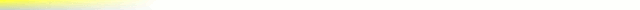# [mkgmap-dev] HGT - getElevation()

From Andrzej Popowski popej at poczta.onet.pl on Fri Jan 19 01:59:14 GMT 2018

```Hi Gerd,

I have prepared patch according to these guessed rules. It rounds
dem-dist and shift coordinates of top-left corner of DEM areas. Since
dem-dist doesn't correspond exactly to HGT, interpolation is always active.

I think there could be 2 ways to improve DEM quality. Interpolation
could be changed to bicubic or mkgmap could use preprocessed data with
correct pixel size. In the latter case probably data format other than
HGT should be used.

--
Best regards,
Andrzej
-------------- next part --------------
Index: src/uk/me/parabola/imgfmt/app/dem/DEMFile.java
===================================================================
--- src/uk/me/parabola/imgfmt/app/dem/DEMFile.java	(revision 4070)
+++ src/uk/me/parabola/imgfmt/app/dem/DEMFile.java	(working copy)
@@ -52,20 +52,57 @@
* @param outsidePolygonHeight the height value that should be used for points outside of the bounding polygon
*/
public void calc(Area area, java.awt.geom.Area demPolygonMapUnits, String pathToHGT, List<Integer> pointDistances, short outsidePolygonHeight) {
-		HGTConverter hgtConverter = new HGTConverter(pathToHGT, area, demPolygonMapUnits);
+		// HGT area is extended by 0.01 degree in each direction
+		HGTConverter hgtConverter = new HGTConverter(pathToHGT, area, demPolygonMapUnits, 0.01D);
hgtConverter.setOutsidePolygonHeight(outsidePolygonHeight);

+		int top = area.getMaxLat() * 256;
+		int bottom = area.getMinLat() * 256;
+		int left = area.getMinLong() * 256;
+		int right = area.getMaxLong() * 256;
+
int zoom = 0;
int lastDist = pointDistances.get(pointDistances.size()-1);
for (int pointDist : pointDistances) {
-			DEMSection section = new DEMSection(zoom++, area, hgtConverter, pointDist, pointDist == lastDist);
+			int distance = pointDist;
+			if (distance == -1) {
+				int res = (hgtConverter.getHighestRes() > 0) ? hgtConverter.getHighestRes() : 1200;
+				distance = (int) ((1 << 29) / (res * 45));
+			}
+			// last 4 bits of distance should be 0
+			distance = ((distance + 8)/16)*16;
+
+			int xtop = top;
+			int xleft = left;
+
+			// align DEM to distance raster, if distance not bigger than widening of HGT area
+			if (distance < (int)Math.floor((0.01D/45.0D * (1 << 29)))) {
+				if (xtop >= 0) {
+					xtop -= xtop % distance;
+					if (xtop < 0x3FFFFFFF - distance)
+						xtop += distance;
+				}
+				else {
+					xtop -= xtop % distance;
+				}
+
+				if (xleft >= 0) {
+					xleft -= xleft % distance;
+				}
+				else {
+					xleft -= xleft % distance;
+					if (xleft > Integer.MIN_VALUE + distance)
+						xleft -= distance;
+				}
+			}
+
+			// to improve: DEM secition should get area in 32bit units
+			DEMSection section = new DEMSection(zoom++, xtop, xleft, xtop - bottom, right - xleft, hgtConverter, distance, pointDist == lastDist);
}
return;
}

-
-
public void write() {
ImgFileWriter w = getWriter();
if (w instanceof BufferedImgFileWriter) {
Index: src/uk/me/parabola/imgfmt/app/dem/DEMSection.java
===================================================================
--- src/uk/me/parabola/imgfmt/app/dem/DEMSection.java	(revision 4070)
+++ src/uk/me/parabola/imgfmt/app/dem/DEMSection.java	(working copy)
@@ -48,16 +48,12 @@
private int maxHeight = Integer.MIN_VALUE;
private List<DEMTile> tiles = new ArrayList<>();

-	public DEMSection(int zoomLevel, Area bbox, HGTConverter hgtConverter, int pointDist, boolean lastLevel) {
+	public DEMSection(int zoomLevel, int areaTop, int areaLeft, int areaHeight, int areaWidth, HGTConverter hgtConverter, int pointDist, boolean lastLevel) {
this.zoomLevel = zoomLevel;
this.lastLevel = lastLevel;

-		if (pointDist == -1) {
-			int res = (hgtConverter.getHighestRes() > 0) ? hgtConverter.getHighestRes() : 1200;
-			pointDist = (int) ((1 << 29) / (res * 45));
-		}
-		this.top = bbox.getMaxLat() * 256;
-		this.left = bbox.getMinLong() * 256;
+		this.top = areaTop;
+		this.left = areaLeft;

// calculate raster that starts at top left corner
// last row and right column have non-standard height / row values
@@ -64,8 +60,8 @@
pointsDistanceLat = pointDist;
pointsDistanceLon = pointDist;

-		int []latInfo = getTileInfo(bbox.getHeight() * 256, pointsDistanceLat);
-		int []lonInfo = getTileInfo(bbox.getWidth() * 256, pointsDistanceLon);
+		int []latInfo = getTileInfo(areaHeight, pointsDistanceLat);
+		int []lonInfo = getTileInfo(areaWidth, pointsDistanceLon);
// store the values written to the header
tilesLat = latInfo;
tilesLon = lonInfo;
===================================================================
@@ -46,14 +46,18 @@
* @param demPolygonMapUnits optional bounding polygon which describes the area for
* which elevation should be read from hgt files.
*/
-	public HGTConverter(String path, Area bbox, java.awt.geom.Area demPolygonMapUnits) {
-		int minLat = (int) Math.floor(Utils.toDegrees(bbox.getMinLat()));
-		int minLon = (int) Math.floor(Utils.toDegrees(bbox.getMinLong()));
-		// the bbox is slightly enlarged so that we dont fail when rounding has no effect
-		// e.g. if getMaxLat() returns 0
-		int maxLat = (int) Math.ceil(Utils.toDegrees(bbox.getMaxLat() + 1));
-		int maxLon = (int) Math.ceil(Utils.toDegrees(bbox.getMaxLong() + 1));
+	public HGTConverter(String path, Area bbox, java.awt.geom.Area demPolygonMapUnits, double extra) {
+		// make bigger box for interpolation or aligning of areas
+		int minLat = (int) Math.floor(Utils.toDegrees(bbox.getMinLat()) - extra);
+		int minLon = (int) Math.floor(Utils.toDegrees(bbox.getMinLong()) - extra);
+		int maxLat = (int) Math.ceil(Utils.toDegrees(bbox.getMaxLat()) + extra);
+		int maxLon = (int) Math.ceil(Utils.toDegrees(bbox.getMaxLong()) + extra);

+		if (minLat < -90) minLat = -90;
+		if (maxLat > 90) maxLat = 90;
+		if (minLon < -180) minLon = -180;
+		if (maxLon > 180) maxLon = 180;
+
minLat32 = Utils.toMapUnit(minLat) * 256;
minLon32 = Utils.toMapUnit(minLon) * 256;